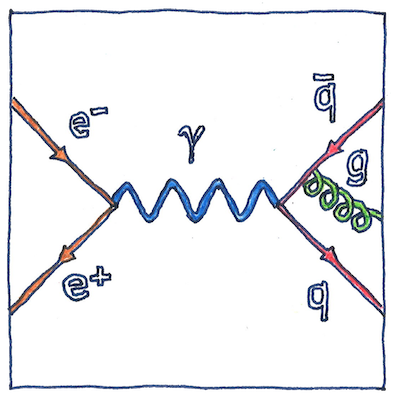# 1948

## The book of science

Tom Sharp

 Richard Feynman quantum mechanics

## Feynman diagram

• A Feynman diagram graphs
• paths of subatomic particles
• against time, in which
• lines entering from the left
• and leaving to the right
• carry energy, momentum, and spin,
• in which each vertex represents
• where particles interact or split,
• and internal lines are propagators.
• Straight lines are fermions,
• wavy lines are bosons,
• and helical lines are gluons.

## Antiparticles seem to go backwards in time

• Ernst Stueckelberg interpreted the positron,
• the electron’s antiparticular mate,
• as though it were an electron moving backward in time.

## Representation

• A Feynman diagram represents
• a possibility for a set of interacting particles,
• but it doesn’t represent all possibilities,
• which would be almost all
• of an infinite number of diagrams,
• which would be like . . . like . . .

One difference between a theorist and an experimentalist in the field of quantum chromodynamics is that the theorist simplifies to essential interactions, when the actual interactions can be far more complex, containing a profusion of gluons sprouting off quarks and antiquarks and decaying into hadrons.

See also in The book of science:

Readings in wikipedia:

Other readings: